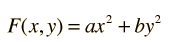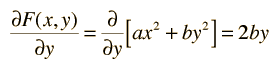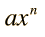# The DerivativeTable:derivatives of functions
Index

Derivative concepts

 HyperPhysics*****HyperMath*****Calculus R Nave
Go Back

# The Partial Derivative

The ordinary derivative of a function of one variable can be carried out because everything else in the function is a constant and does not affect the process of differentiation. When there is more than one variable in a function it is often useful to examine the variation of the function with respect to one of the variables with all the other variables constrained to stay constant. This is the purpose of a partial derivative. The derivative is carried out in the same way as ordinary differentiation with this constraint. For example, given the polynomial in variables x and y,the partial derivative with respect to x is writtenand the partial derivative with respect to y is writtenIndex

Derivative concepts

 HyperPhysics*****HyperMath*****Calculus R Nave
Go Back

# Time Derivatives

Time derivatives are the standard way of representing instantaneous velocities and accelerations.One example of the use of the derivative is in obtaining the velocity and acceleration from a position equation.

Index

Derivative concepts

 HyperPhysics*****HyperMath*****Calculus R Nave
Go Back

# Derivatives of Polynomials

Many functions in physical problems have the form of polynomials. The derivative of a polynomial is the sum of the derivatives of its terms, and for a general term of a polynomial such asthe derivative is given byOne of the common applications of this is in the time derivatives leading to the constant acceleration motion equations.

Index

Derivative concepts

 HyperPhysics*****HyperMath*****Calculus R Nave
Go Back